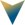# The Chemistry of Asset Allocation

Risk factors as building blocks for portfolio diversification: The chemistry of Asset Allocation

Because seemingly diverse asset classes may have high correlations as a result of overlapping risk factor exposures, factor analysis can improve portfolio diversification. A factor based approach enables the investor theoretically to remix the factors into portfolios that are better diversified and more efficient than traditional portfolios. Although it is possible to have ex ante factor optimized portfolios, there are challenges to overcome such as the need for active, frequent rebalancing, creation of forward looking assumptions, and use of derivatives and short positions.

What is an asset class?

Asset classes are bundles of risk exposures divided into categories – such as equity, debt and real assets – based on their financial characteristics. Ideally, asset classes are as independent as possible, with little overlap and, in aggregate, cover the investment universe with minimal gaps.

Modern Portfolio Theory

One of the key insights of MPT is that correlations less than 100% lead to diversification benefits. Thus, asset classes with low correlations can together create an efficient portfolio. Efficiency is derived by optimally allocating various asset classes in a portfolio. The most efficient portfolio is the one which provides the greatest expected return for a given level of expected risk. Risk expectation is set using the desired exposures to various risk factors.

Diversification in name only:

When a portfolio appears to have been diversified across asset classes but those asset classes have high correlations, the portfolio’s diversification is said to be in the name only. For example, at the outset, bonds and equity are vastly different but US equity and US corporate bonds share some common risk exposures such as currency, volatility and inflation risk exposures. Thus, decomposing the portfolio into factor exposures broadens our understanding of the relationship between asset classes.

Note that macroeconomic factors are applicable to most asset classes whereas equity and fixed income deconstruct characteristics within those two broad asset classes. Some types of factors are liquidity, leverage and private markets. Developed economy, as a factor, packs together GDP growth, productivity, liquidity along with other characteristics. Cash would be a combination of real interest rates and inflation. Investors can gain exposure to factors via investable proxies, although some factors are easier to access than others.

For some factors, there is no natural way to invest, while for some there are ways:

• Inflation: Long a nominal Treasuries index, short a Treasury Inflation Protected Securities (TIPS) index
• Real interest rates: Long a TIPS index
• Volatility: Long volatility futures
• Value: Long an equity value index, short an equity growth index
• Size: Long an equity small-cap index, short an equity large cap index
• Credit spread: Long a high quality credit index, short a Gilt index

Return, risk and correlation

The correlation relationships exhibit greater stability over time than return and standard deviation do. In the illustration shown in this paper, the factor portfolio with equal weighted exposure to 10 factors and monthly rebalancing has 34 units of variance compared with the 119 units in the traditional portfolio over 15 years. The factor portfolio achieved a slightly higher level of return than the traditional portfolio while taking on about one quarter of the volatility.

Factor characteristics appear to be time-period dependent; if different start or end dates were selected, both factor and traditional portfolios would have different risk and return characteristics. However, a factor portfolio can have fundamentally diverse characteristics from a traditional asset class portfolio – and also less volatility.

A portfolio manager’s skill is tested while building an optimized portfolio – allocating weights to factors in a manner that yield maximum returns. Back-testing can show which factors have worked to what extent in the past. The same could be extrapolated to the future but with caution – skills, thus, become important.

Challenges to factor based investing in practising

• No theoretical set encompasses all of the significant factors
• Many factors, such as global GDP growth and momentum, have poor investible proxies
• Most academic studies use equal-weight portfolios while practically, optimized portfolios are needed
• Frequent and attentive rebalancing is needed to maintain the desired factor exposures over time. This comes at a cost, primarily transaction cost
• Policy level or regulatory restrictions might disallow or limit investment in certain asset classes such as derivatives and some alternatives

De-risking: Factor-based approaches are conducive to attenuating common sources of risk in traditional portfolios—that is, de-risking. For instance, the prevalence of risk stemming from equity can be reduced by introducing factors such as those under the macroeconomic and fixed income factors. Additionally, one can readily incorporate liability-driven investing (LDI) by treating the liability as an asset held short and allocating appropriate weights to interest rate, duration, inflation, credit spread, and other factors that mimic the liability profile. Such an approach could also incorporate the credit exposure essential to hedging liabilities discounted by corporate bond curves.